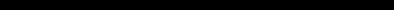# ZX 81 - BASIC ProgrammingINDEX Front Cover Chapter 1 Setting up the ZX81 Chapter 2.1 Telling the computer what to do 1 Chapter 2.2 Telling the computer what to do 2 Chapter 3 A History lesson Chapter 4.1 The Sinclair ZX81 as a calculator Chapter 4.2 The Sinclair ZX81 as a calculator - Exercises Chapter 5.1 Functions Chapter 5.2 Functions - Exercises Chapter 6.1 Variables Chapter 6.2 Variables - Exercises Chapter 7.1 Strings Chapter 7.2 Strings - Exercises Chapter 8.1 Computer programming Chapter 8.2 Computer programming - Exercises Chapter 9.1 More computer programming Chapter 9.2 More computer programming - Exercises Chapter 10.1 If... Chapter 10.2 If... - Exercises Chapter 11.1 The character set Chapter 11.2 The character set - Exercises Chapter 12.1 Looping Chapter 12.2 Looping - Exercises Chapter 13 Slow & fast Chapter 14.1 Subroutines Chapter 14.2 Subroutines - Exercises Chapter 15.1 Making your programs work Chapter 15.2 Making your programs work - Exercises Chapter 16.1 Tape storage Chapter 16.2 Tape storage - Exercises Chapter 17.1 Printing with frills Chapter 17.2 Printing with frills - Exercises Chapter 18.1 Graphics Chapter 18.2 Graphics - Exercises Chapter 19.1 Time & motion Chapter 19.2 Time & motion - Exercises Chapter 20.1 The ZX Printer Chapter 20.2 The ZX Printer - Exercises Chapter 21.1 Substrings Chapter 21.2 Substrings - Exercises Chapter 22.1 Arrays Chapter 22.2 Arrays - Exercises Chapter 23 When a computer gets full Chapter 24.1 Counting on your fingers Chapter 24.2 Counting on your fingers - Exercises Chapter 25 How the computer works Chapter 26.1 Using machine code Chapter 26.2 Using machine code - Exercises Chapter 27 Organization of memory Chapter 28.1 The system variables Chapter 28.2 The system variables - Exercises Appendix A The character set Appendix B Report codes Appendix C.1 The ZX81 for those that understand BASIC 1 Appendix C.2 The ZX81 for those that understand BASIC 2 Appendix C.3 The ZX81 for those that understand BASIC 3